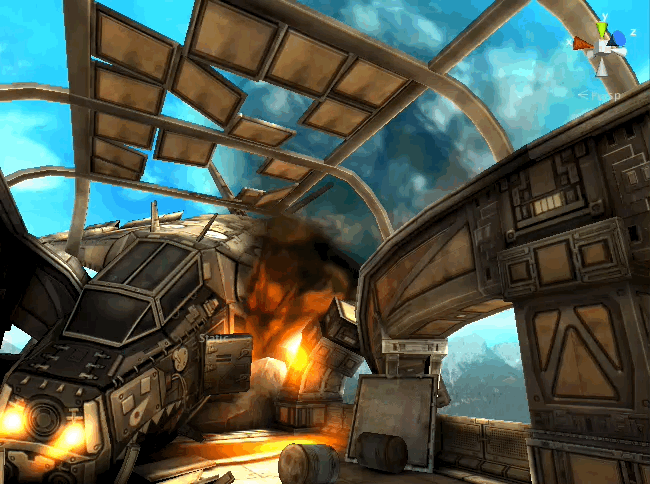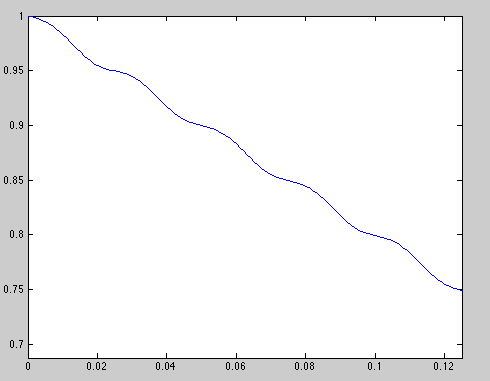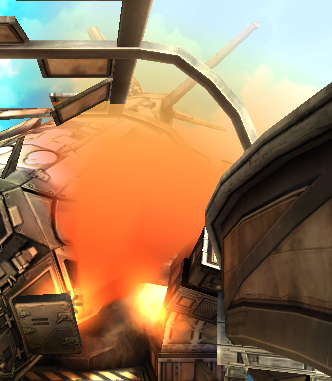# ShadowGun

ShadowGun其实最开始是2011年的一个移动平台的第三人称射击游戏。当然，也是用Unity开发的。当年，由于在画面上的出色表现赢得了很多眼球~更难能可贵的是，在2012的时候，它的开发者放出了示例场景，来让更多的开发者学习如何优化移动平台上的shader。下载地址请戳官方博客。看不懂英文的可以看这篇（写得很不错）。项目里共包含了将近20个优化后的shader。关于使用许可的问题，项目里的shader都是可以免费使用的，而贴图和模型是不可用于商业用途的呦~

ShadowGun里包含了几个比较重要的shader，例如非常有名的旗帜飘动的shader，动态效果的天空盒子的shader，环境高光纹理映射等等。而这篇的飞机坠毁的浓烟效果shader应该是其中非常好理解的一篇。我们也从这里开始学习。

# 浓烟效果# Shader源码

Shader "MADFINGER/Environment/Scroll 2 Layers Sine AlphaBlended" {
Properties {
_MainTex ("Base layer (RGB)", 2D) = "white" {}
_DetailTex ("2nd layer (RGB)", 2D) = "white" {}
_ScrollX ("Base layer Scroll speed X", Float) = 1.0
_ScrollY ("Base layer Scroll speed Y", Float) = 0.0
_Scroll2X ("2nd layer Scroll speed X", Float) = 1.0
_Scroll2Y ("2nd layer Scroll speed Y", Float) = 0.0
_SineAmplX ("Base layer sine amplitude X",Float) = 0.5
_SineAmplY ("Base layer sine amplitude Y",Float) = 0.5
_SineFreqX ("Base layer sine freq X",Float) = 10
_SineFreqY ("Base layer sine freq Y",Float) = 10
_SineAmplX2 ("2nd layer sine amplitude X",Float) = 0.5
_SineAmplY2 ("2nd layer sine amplitude Y",Float) = 0.5
_SineFreqX2 ("2nd layer sine freq X",Float) = 10
_SineFreqY2 ("2nd layer sine freq Y",Float) = 10
_Color("Color", Color) = (1,1,1,1)

_MMultiplier ("Layer Multiplier", Float) = 2.0
}

SubShader {
Tags { "Queue"="Transparent" "IgnoreProjector"="True" "RenderType"="Transparent" }

Blend SrcAlpha OneMinusSrcAlpha
Cull Off Lighting Off ZWrite Off Fog { Color (0,0,0,0) }

LOD 100

CGINCLUDE
#pragma multi_compile LIGHTMAP_OFF LIGHTMAP_ON
#pragma exclude_renderers molehill
#include "UnityCG.cginc"
sampler2D _MainTex;
sampler2D _DetailTex;

float4 _MainTex_ST;
float4 _DetailTex_ST;

float _ScrollX;
float _ScrollY;
float _Scroll2X;
float _Scroll2Y;
float _MMultiplier;

float _SineAmplX;
float _SineAmplY;
float _SineFreqX;
float _SineFreqY;

float _SineAmplX2;
float _SineAmplY2;
float _SineFreqX2;
float _SineFreqY2;
float4 _Color;

struct v2f {
float4 pos : SV_POSITION;
float4 uv : TEXCOORD0;
fixed4 color : TEXCOORD1;
};

v2f vert (appdata_full v)
{
v2f o;
o.pos = mul(UNITY_MATRIX_MVP, v.vertex);
o.uv.xy = TRANSFORM_TEX(v.texcoord.xy,_MainTex) + frac(float2(_ScrollX, _ScrollY) * _Time);
o.uv.zw = TRANSFORM_TEX(v.texcoord.xy,_DetailTex) + frac(float2(_Scroll2X, _Scroll2Y) * _Time);

o.uv.x += sin(_Time * _SineFreqX) * _SineAmplX;
o.uv.y += sin(_Time * _SineFreqY) * _SineAmplY;

o.uv.z += sin(_Time * _SineFreqX2) * _SineAmplX2;
o.uv.w += sin(_Time * _SineFreqY2) * _SineAmplY2;

o.color = _MMultiplier * _Color * v.color;
return o;
}
ENDCG

Pass {
CGPROGRAM
#pragma vertex vert
#pragma fragment frag
#pragma fragmentoption ARB_precision_hint_fastest
fixed4 frag (v2f i) : COLOR
{
fixed4 o;
fixed4 tex = tex2D (_MainTex, i.uv.xy);
fixed4 tex2 = tex2D (_DetailTex, i.uv.zw);

o = tex * tex2 * i.color;

return o;
}
ENDCG
}
}
}


# SubShader Tags

Tags { "Queue"="Transparent" "IgnoreProjector"="True" "RenderType"="Transparent" }

# 渲染设置

	Blend SrcAlpha OneMinusSrcAlpha
Cull Off
Lighting Off
ZWrite Off
Fog { Color (0,0,0,0) }

Cull Off 指明该shader不会剔除面，即背面也会被渲染。

ZWrite Off 指明不写入深度缓存，而是根据渲染顺序来决定显示的。其实"Queue"="Transparent"会自动生成ZWrite Off 语句的。

# 算法分析

	v2f vert (appdata_full v)
{
v2f o;
o.pos = mul(UNITY_MATRIX_MVP, v.vertex);
o.uv.xy = TRANSFORM_TEX(v.texcoord.xy,_MainTex) + frac(float2(_ScrollX, _ScrollY) * _Time);
o.uv.zw = TRANSFORM_TEX(v.texcoord.xy,_DetailTex) + frac(float2(_Scroll2X, _Scroll2Y) * _Time);

o.uv.x += sin(_Time * _SineFreqX) * _SineAmplX;
o.uv.y += sin(_Time * _SineFreqY) * _SineAmplY;

o.uv.z += sin(_Time * _SineFreqX2) * _SineAmplX2;
o.uv.w += sin(_Time * _SineFreqY2) * _SineAmplY2;

o.color = _MMultiplier * _Color * v.color;
return o;
}

u = sin(freq * x) * ampl + scroll * x;Shader利用了两种纹理来模拟立体效果，每张纹理对应了6个参数，分别代表了U方向和V方向上的函数参数。o.color = _MMultiplier * _Color * v.color;

Shader允许我们在面板中（通过_MMultiplier_Color）在顶点颜色的基础上调整整体颜色，并将结果存储到v2f中。要注意的是，这里的顶点颜色，即v.color，包含了重要的透明度信息，浓烟的透明过渡效果其实都是它的功劳。

# 写在最后